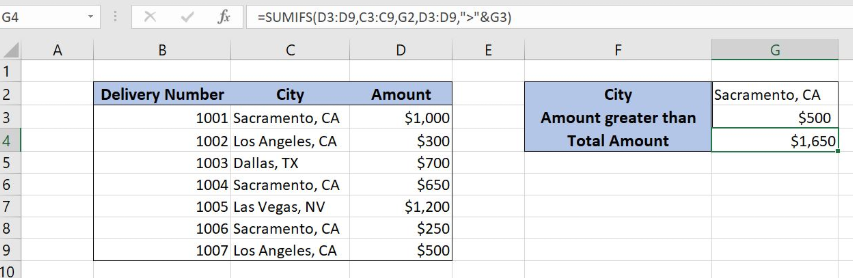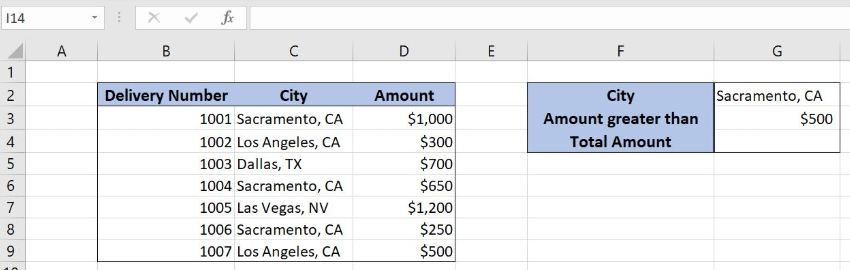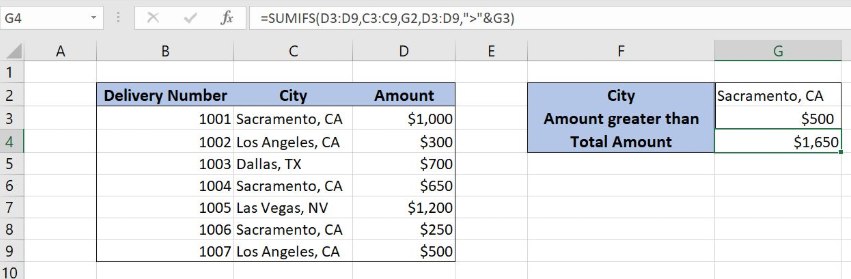Get instant live expert help with Excel or Google Sheets“My Excelchat expert helped me in less than 20 minutes, saving me what would have been 5 hours of work!”

#### Post your problem and you'll get expert help in seconds

Your message must be at least 40 characters
Our professional experts are available now. Your privacy is guaranteed.

# Sum if Multiple Criteria

Excel allows us to sum all values from a table that meet multiple criteria using the SUMIFS function. This step by step tutorial will assist all levels of Excel users in summing values if multiple criteria are met.Figure 1. The final result of the SUMIFS function

## Syntax of the SUMIFS formula

`=SUMIFS(sum_range, criteria_range1, criteria1, criteria_range2, criteria2)`

The parameters of the SUMIFS function are:

• sum_range – a range with values which we want to sum
• criteria_range1 – a range where we want to set our first condition
• criteria1 – the first condition for summing the values
• criteria_range2 – a range where we want to set our second condition
• criteria2 – the second condition for summing the values

## Setting up Our Data for the SUMIFS Function

Our table consists of 3 columns: “Delivery Number” (column B), “City” (column C) and “Amount” (column D). In the cell G2, we specify a value that we want “City” column to contain. In G3 we specify an amount that we want “Amount” column to be greater than. In the cell G4, we want to get a sum for these two conditions.Figure 2. Data that we will use in the SUMIFS example

## Sum Amount if Multiple Criteria are True

In our example, we want to sum all amounts from column D if the cell in column C contains “Sacramento CA”. And value in column D is greater than \$500.

The formula looks like:

`=SUMIFS(D3:D9, C3:C9, G2, D3:D9, ">"&G3)`

The sum_range is D3:D9. The criteria_range1 is C3:C9 (“City” column), while the criteria1 is G2 (Sacramento, CA). The criteria_range2 is D3:D9 (“Amount” columns) and the criteria2 is “>”&G3. We want to get all values greater than G3, that’s why we need to put “>”& before G2.

To apply the SUMIFS function, we need to follow these steps:

• Select cell G4 and click on it
• Insert the formula: `=SUMIFS(D3:D9,C3:C9,G2,D3:D9,">"&G3)`
• Press enterFigure 3. Using the SUMIFS function to sum values that meet multiple criteria

In this example, we get all the amounts which have “Sacramento, CA” in “City” and the amount greater than \$500 in “Amount”. As you can see, rows 1 and 6 meet both conditions, so correspondings amounts are summed (\$1,000 and \$650). Finally, the sum in the cell G4 is \$1,650.

Most of the time, the problem you will need to solve will be more complex than a simple application of a formula or function. If you want to save hours of research and frustration, try our live Excelchat service! Our Excel Experts are available 24/7 to answer any Excel question you may have. We guarantee a connection within 30 seconds and a customized solution within 20 minutes.

### Did this post not answer your question? Get a solution from connecting with the expert.Another blog reader asked this question today on Excelchat:## Subscribe to Excelchat.coAnother blog reader asked this question today on Excelchat: